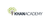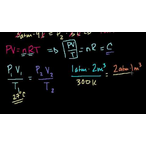# Class 11 Physics (India): Ideal gas equation example 2

Type product
Tijdsduur

## Class 11 Physics (India): Ideal gas equation example 2Khan Academy

Tip: laat jezelf inspireren, bekijk hier de video!### Beschrijving

PV/T is a constant. Figuring out the volume of an ideal gas at standard temperature and pressure (STP).

## Class 11 Physics (India)

Let's learn, practice, and master topics of class 11 physics (NCERT) starting with kinematics and then moving to dynamics with Newton's laws of motion, work, energy, and power. Let's then use these as the foundation to learn about centre of mass, rotational motion, gravitation, solids, fluids, thermodynamics, and oscillations and waves.

## Ideal gas equation

In this tutorial, Sal shows you how to use the ideal gas equation in calculations.

## Topic: Science

Learn about all the sciences, from physics, chemistry and biology, to cosmology and astronomy, across hundreds of v…

Lees de volledige beschrijving

### Veelgestelde vragen

Er zijn nog geen veelgestelde vragen over dit product. Als je een vraag hebt, neem dan contact op met onze klantenservice.

Nog niet gevonden wat je zocht? Bekijk deze onderwerpen: Natuurkunde, Biologie, Chemie, Wildbeheer en Scala.

PV/T is a constant. Figuring out the volume of an ideal gas at standard temperature and pressure (STP).

## Class 11 Physics (India)

Let's learn, practice, and master topics of class 11 physics (NCERT) starting with kinematics and then moving to dynamics with Newton's laws of motion, work, energy, and power. Let's then use these as the foundation to learn about centre of mass, rotational motion, gravitation, solids, fluids, thermodynamics, and oscillations and waves.

## Ideal gas equation

In this tutorial, Sal shows you how to use the ideal gas equation in calculations.

## Topic: Science

Learn about all the sciences, from physics, chemistry and biology, to cosmology and astronomy, across hundreds of videos, articles and practice questions.

Er zijn nog geen ervaringen.
• Bekijk gerelateerde producten mét ervaringen: Natuurkunde.

### Deel je ervaring

Heb je ervaring met deze cursus? Deel je ervaring en help anderen kiezen. Als dank voor de moeite doneert Springest € 1,- aan Stichting Edukans.

Er zijn nog geen veelgestelde vragen over dit product. Als je een vraag hebt, neem dan contact op met onze klantenservice.